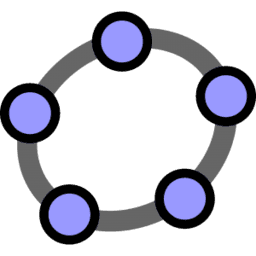# Learn Calculator Suite

Welcome to the GeoGebra Calculator Suite! Learn how to use this full powered math app including function graphing, tables, equation solving, derivatives and integrals, statistics, dynamic geometric constructions and more:
• Plot functions, implicit equations, polar and parametric curves
• Experience transformations with sliders
• Build tables of values
• Find special points of functions: roots, min, max, intersections
• Solve equations
• Find derivatives and integrals
• Construct and analyze many types of regressions
• Create geometric constructions
• Measure lengths, perimeters, and areas
• Create and analyze traces of points and loci
• Investigate construction steps to get a deeper understanding
• Save and share your results with students and teachers
GeoGebra Calculator Suite is the successor of our good old GeoGebra Classic app, so we will include all the great features you love in this app and add even more in the future!